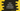# Dart tutorial : How to use Boolean in Dart### How to use Boolean in Dart :

Dart comes with a ‘Boolean’ datatype for variables. The value of Boolean is either true or false. You cannot assign any other values to Booleans. Keyword bool is used to define a boolean variable. For example, let’s take a look at the program below :

``````main(){
bool first = false;
bool second = true;

if(first){
print("first is true");
}else{
print("first is false");
}

if(second){
print("second is true");
}else{
print("second is false");
}
}``````

It will print the below output :

``````first is false
second is true``````

This is a basic example of using boolean in dart. We have assigned one variable the value of true and false to the other. But what will happen if we assign a different value to a boolean variable? Let’s check :

``````main(){
bool first = 1;

if(first){
print("first is true");
}else{
print("first is false");
}
}``````

Output :

``first is false``

So, the value of first is false, i.e. if we assign any other values to a boolean, it will be false.If we try to run it in checked mode, it will throw an error. So, if you are using boolean, always assign it to value of true or false.

### Where we are using boolean :

Boolean is returned by many functions. If we are using any such methods, we can use them with if-else conditions.For example,checking for a null value of a variable, checking the empty condition of a string etc. Let’s try to understand with an example :

``````main(){
var myString = "Hello World";
var myNumber = 2;
var myVariable = null;

if(myString.isEmpty){
print("myString is empty");
}else{
print("myString is not empty");
}

if(myNumber < 10){
print("myNumber is less than 10");
}else{
print("myNumber is greater than 10");
}

if(myVariable == null){
print("myVariable is null");
}else{
print("myVariable is not null");
}
}``````

Output :

``````myString is not empty
myNumber is less than 10
myVariable is null``````

Here, we are using three if-else statements. The first statement checks if a string is empty or not, the second statement checks if a number is less than 10 or not and the third statement checks if a variable is null or not. If condition we are using inside if codition is a boolean value.It is either true or false.

### Conclusion :

So, we have learnt that boolean in dart either holds one true or false value, if we assign any other value than these, it will automatically become false.Go through the above examples and drop one comment if you have any queries.